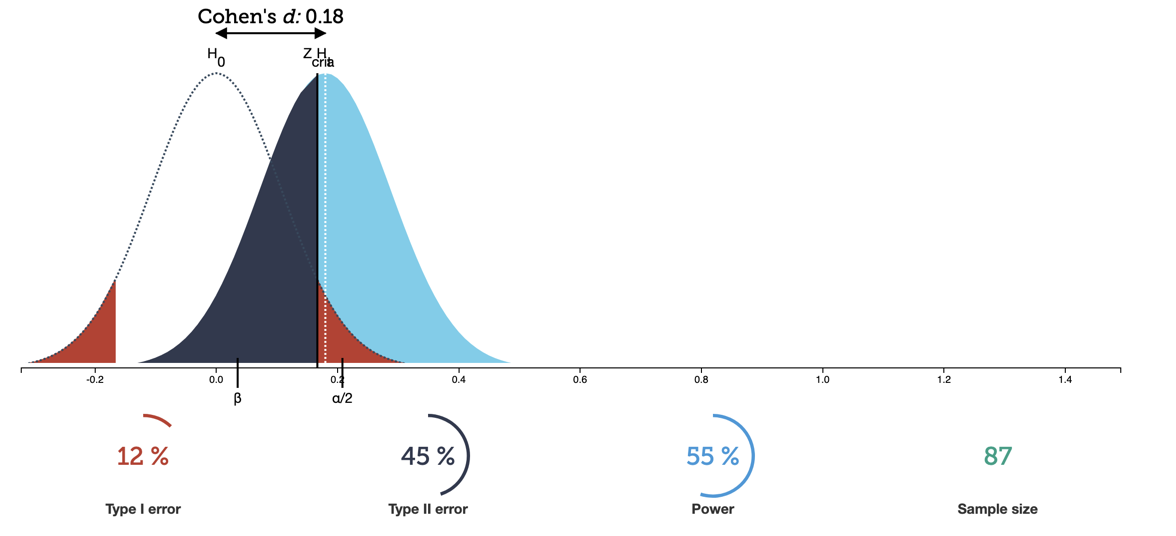# AB Testing

https://hbr.org/2017/06/a-refresher-on-ab-testing

https://blog.minitab.com/blog/alphas-p-values-confidence-intervals-oh-my

## Decide what you want to test

Your test needs to perform better or worse at something measurable. Ex: The number of users who click on a button.

## Statistical significance

P values are the probability of observing a sample statistic that is at least as extreme as your sample statistic when you assume that the null hypothesis is true.

High p-values indicate that your evidence is not strong enough to suggest an effect exists in the population. An effect might exist but it’s possible that the effect size is too small, the sample size is too small, or there is too much variability for the hypothesis test to detect it.

## Type I,II Errors

Type I: believing there is an effect when there is actually no effect

a type I error is the rejection of a true null hypothesis (also known as a "false positive" finding or conclusion; example: "an innocent person is convicted")

• To reduce Type I error, increase sample size, increase effect size,

α (Alpha) is the probability of a type I error.

confidence level + alpha = 1: Lower alpha means higher confidence level.

P Value vs alpha: The P Value is what your experiment shows. If the P Value is greater than Alpha, you accept the null hypothesis (that there is no effect). If the P Value is lower than Alpha, you reject the null hypothesis.

Ex: You set an alpha of 0.05. Your confidence level becomes 0.95. Let’s say your test returns a P Value of 0.03, it’s less than alpha (0.05), so you reject the null hypothesis and say there was an effect. If you are wrong, that is a Type I error.

Type II: believing there is no effect when there is actually an effect

while a type II error is the non-rejection of a false null hypothesis (also known as a "false negative" finding or conclusion; example: "a guilty person is not convicted")

β (Beta) is the probability of Type II error

Power is `1-β`, when high it means there is a low probability of type II error

Ex: You set an alpha of 0.03. Your confidence level becomes 0.97. Let’s say your test returns a P Value of 0.4, it’s greater than alpha (0.03), so you accept the null hypothesis and say there was no effect. If you are wrong, that is a type II error.

If the effect you are measuring may be small, then you must aim to increase your sample size or you are likely to encounter a type II error.

If your sample size may be small, then you must aim to increase to your effect size or you are likely to encounter a type II error as well.

Why? Because it’s hard to prove that highly overlapping distributions are different distributions and not the same distribution.## Blocking

Look for variables that may not be easily manipulated, but have an effect on the outcome. For example, users on mobile vs. Desktop might have different click rates on buttons. Ensure data is split into groups in these cases, showing the relative effect on mobile and desktop users separately.

## Multivariate tests

You should run more complex tests all at once rather than sequential tests. This is so that you don’t miss winning combination after excluding possibilities eliminated by previous tests.

## Example report

“Control: 12% (+/- 2.1%) Variation 15% (+/- 2.3%).” With a 95% confidence interval (5% alpha) means that 95% of future experiments would contain the true value (not that there is a 95% chance of this particular range containing the true value)